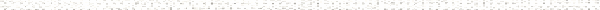A Precalculus OutlineObjective: Correctly define a set.Objective: Given two sets, write the set representing their intersection.Objective: Given two sets, write the set representing their union.Objective: Given ordered pairs, plot and label points using the cartesian coordinate system.Objective: Given an ordered pair, identify the quadrant in or axis on which the point lies.Objective: Given an ordered pair, write the ordered pair of the point symmetric to the given point about the x-axis, the y-axis, and the origin.Objective:  Correctly write and use the distance formula to find the exact distance between two given points.Objective: Correctly write and use the midpoint formula to find the midpoint of the line segment connecting two given points.Objective: Apply the distance formula and/or midpoint formula to solve applications involving distance and midpoint.Objective: Describe a set using a sentence by the verbal method.Objective: Describe a set using the roster method.Objective: Describe a set using the set-builder method.Objective: Define the empty set.Objective: Define and write examples of each set: natural numbers, whole numbers, integers, rational numbers, real numbers, irrational numbers, and complex numbers.Objective: Apply interval notation to write an inequality as an interval.Objective: Given an inequality, graph an inequality region on the real number line.Objective: Given an interval, graph an inequality region on the real number line.Objective: Graph points on a real number line.Objective: Apply the quotient rule for radicals.Objective: Simplify sums and differences of radical terms.Objective: Simplify the products of radicals.Objective: Write each product of radicals as a single radical.Objective: Simplify complex fractions.Objective: Graph simple inequalities on a real number line.Objective: Graph compound inequalities on a real number line.Objective: Identify and name the quadrants, the origin, and the axes relating to the cartesian coordinate system.Objective: Demonstrate the concept of symmetry.Objective: Correctly state and apply the pythagorean theorem.Objective: Evaluate radical expressions.Objective: Simplify radical expressions.Objective: Apply the product rule for radicals.Objective: Given a relation as a set of ordered pairs, graph the relation using the cartesian coordinate system.Objective: Given an equation, analytically test the graph for symmetry with respect to the y-axis.Objective: Given an equation, analytically test the graph for symmetry with respect to the origin.Objective: Given a relation as an infinite set of points, graph the relation using the cartesian coordinate system.Objective: Given a graph of a relation, describe the graph using the roster method.Objective: Given an equation, graph a horizontal line.Objective: Given an equation, graph a vertical line.Objective: Given an equation, write ordered pairs for any x-intercept(s) and for any y-intercept(s).Objective: Given an equation, construct a table of ordered pairs that satisfy the equation.Objective: Given an equation, create a rough sketch of the graph of the equation.Objective: Given an equation, analytically test the graph for symmetry with respect to the x-axis.Objective: Graphing Linear Inequalities in One Variable in the Plane.Objective: Given ordered pairs, plot points using the cartesian coordinate system.Objective: Describe and demonstrate symmetry with respect to the origin.Objective: Given an equation, substitute (-y) for y and simplify the result.Objective: Given an equation, substitute (-x) for x and simplify the result.Objective: Given an equation, substitute (-y) for y and substitute (-x) for x and simplify the result.Objective: Correctly read and describe an inequality.Objective: Graph an inequality on a line number line.Objective: Describe the equation for the x-axis and the equation for the y-axis.Objective: Solve an equation for a specified variable.Objective: Complete a table of ordered pairs that satisfy a given equation.Objective: Using a table of ordered pairs, accurately plot and correctly connect points to sketch the graph of an equation.Objective: Describe and demonstrate symmetry with respect to the x-axis.Objective: Describe and demonstrate symmetry with respect to the y-axis.Objective: Given sets of ordered pairs, determine which relations represent y as a function of x.Objective: Given a set of ordered pairs that represents a function, write the domain and range of the function.Objective: Given graphs of relations, use the vertical line test to determine which graphs represent y as a function of x.Objective: Given a graph that represents a function, write the domain and range of the function.Objective: Given equations, determine which equations represent y as a function of x.Objective: Given a list of ordered pairs, accurately plot points.Objective: Correctly read and interpret graphs with open and/or closed endpoints.Objective: Given an equation, write the equation in an equivalent form by solving for y.